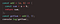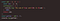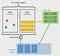# Async Nature of JavaScript

If you are new to JavaScript then you must have heard that JavaScript is an asynchronous language but what if I say you are wrong JavaScript is not asynchronous it is a synchronous language, then why is everyone saying it is Async. Let’s read the below article to understand.

# What is Synchronous JavaScript?

When you write a piece of code and each code of line executes one after another, then it is a synchronous. Each step of operation waits for the previous step to execute completely. Let’s see an example below to understand this.Fig 1. A synchronous function to calculate the sum of two number

In the Fig 1, let’s see how the code works. The code is executed as below:

1. The first line of code which will execute is getSum=add(2,3).
2. This will call the function add in the first line.
3. Then the sum of a and b is stored in constant variable sum.
4. Then this sum is returned and it stores the value in const variable getSum
5. Then the getSum is printed at last.

In the above we can observe that console.log is waiting for the sum to be returned. This is because JavaScript is a single threaded, only one thing can happen at single point of time in the single thread, hence it is waiting for the previous step to execute until then everything is blocked.

# What is Asynchronous JavaScript?

When you write a piece of code and a particular step doesn’t wait for the previous step to execute completely, then it is asynchronous. This happens when a API is called which uses external device to get the data, then it doesn’t make sense to block the entire execution until the data comes and then to execute the next steps. Let’s see an example below to understand.Fig 2. An asynchronous function setTimeout which prints the sum after 3s.

In the Fig 2, what do you think will be the output of the above code? Will the ‘The sum of 2 and 3 is 5’ print first followed by value of sum or will it print later. Let’s see how the code works, the code is executed as below:

1. The first line of code which will execute is getSum=add(2,3).
2. This will call the function add in the first line.
3. Then the sum of a and b is stored in constant variable sum.
4. Now the setTimeout doesn’t run immediately, it is sent to the browser to execute that, once it is executed the callback function is sent to event queue(will discuss about this later).
5. Then this sum is returned and it stores the value in const variable getSum
6. Then the getSum is printed at last.
7. Now when the call stack gets empty then the callback function comes to the main thread and will print ‘The sum of 2 and 3 is 5’ after 3s

So what happened above, why ‘The sum of 2 and 3 is 5’ printed after ‘5’ even when it was called before. It is because we have a concept of event queue and event loop which executes the async calls only after the call stack is empty.

# What is Event Queue and Event Loop?

Async calls are put into event queue or callback queue, which runs after the call stack becomes empty i.e after the main thread has finished processing all the operations, so that these async calls do not block the next javascript codes from running.Fig 3. How Event loop and Callback Queue works in JS Engine

In the Fig 3, these callback queues are sent back to the main thread once all the codes are executed inside it and then these queued codes are executed. The event queues are sent back to the main thread with the help of event loop. The event loop continuously runs and check if the main thread has become empty or not, as soon as the main thread becomes empty the queues are pushed to the call stack.

# Conclusion

As we read above, it proves JS is a synchronous, blocking and single threaded language. It is the external API’s which make the language behave asynchronous and to overcome these there is a concept of event queue and event loop. These things are controlled by JavaScript engine and not the Javascript itself. The browsers have the engine, V8 is one of the most used engine.